# 1766 A Polygonal Elephant for World Elephant Day

### Today’s Puzzle:

Today many people are reflecting on the plight of elephants throughout the world and what we can do to protect them and their habitats. Yesterday I created an image of a lone elephant in Desmos: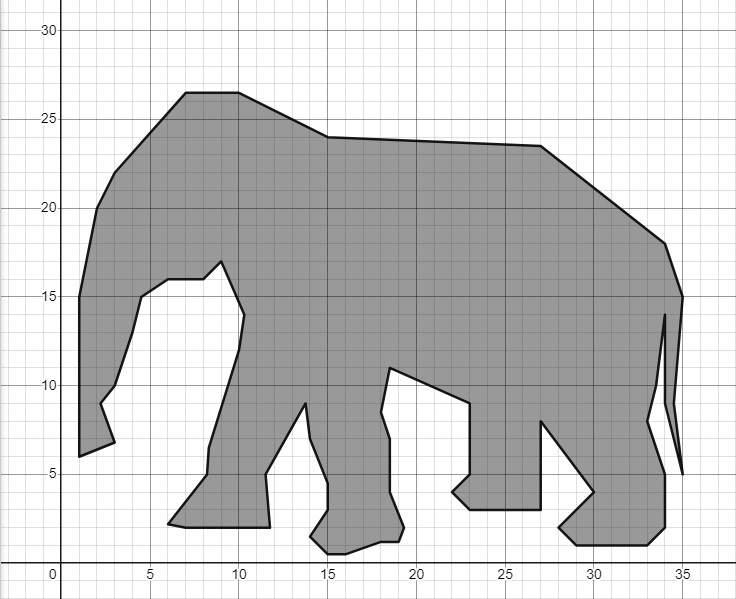Today’s puzzle is to take the Desmos Elephant image and transform it as I have done below. There is no need to retype all the ordered pairs that made the elephant. One of the reflections was made simply by typing “polygon(x1,-y1)” below all the order pairs in the Desmos image. Can you determine which reflection that was? What was typed inside the parenthesis to make the other two elephants?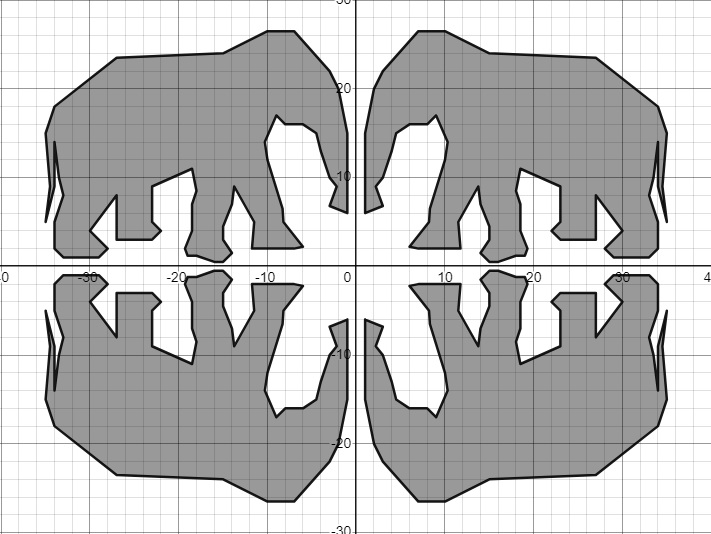How would the elephant be transformed if you typed “polygon(-y1,x1)”, “polygon(x1+5,y1)” or  “polygon(2×1,2y1)” below the ordered pairs in Desmos? Can you figure out a way to shrink the elephant and make it upside down in the first quadrant? I hope you will take the time to check out these transformations and experiment with some of your own.

### Factors of 1766:

• 1766 is a composite number.
• Prime factorization: 1766 = 2 × 883.
• 1766 has no exponents greater than 1 in its prime factorization, so √1766 cannot be simplified.
• The exponents in the prime factorization are 1 and 1. Adding one to each exponent and multiplying we get (1 + 1)(1 + 1) = 2 × 2 = 4. Therefore 1766 has exactly 4 factors.
• The factors of 1766 are outlined with their factor pair partners in the graphic below.### More About the Number 1766:

1766 looks interesting in some other bases:
It’s 6E6 in base 16 because 6(16²) + 14(16) + 6(1) = 1766,
272 in base 28 because 2(28²) + 7(28) + 2(1) = 1766, and
123 in base 41 because 1(41²) + 2(41) + 3(1) = 1766.

# 1764 Perfect Squares Are Amazing!

### Today’s Puzzle:

1764 is a perfect square, and its last two digits are a perfect square, too. The same thing was true of the last perfect square, 1681. And it will be true of the next perfect square, 1849. These perfect squares got me curious about perfect squares in general, and I noticed something. Can you notice it, too?

Look at this graphic of perfect squares. What do you notice? What do you wonder?I have known for years that the last digits of perfect squares follow a palindromic
0-1-4-9-6-5-6-9-4-1-0 pattern. The first time the backward repeating happens is at 5¹.

I had no idea that the last two digits followed a palindromic pattern, too! Notice that the first time the backward repeating of the last two digits happens is at (5²)² or 25². It also happens at 75², 125², and so forth. The forward repeating happens at 0², 50², 100², and so forth. Note that (-n)² = n², so this pattern goes on in both directions FOREVER!

Also if the last two digits of a number aren’t either 00, 25, or (even number)1, (even number)4, (even number)9,  or (odd number)6, then no way is it a perfect square!

I wondered if the last three digits would backward repeat at (5³)² or 125². It doesn’t. 🙁
But at 250², it DOES! Rather than make a graphic with all 250 rows, I just made one with the last 25 rows:Will the last FOUR numbers start backward repeating at 2500²? I know the answer, but perhaps you would like to find out for yourself!

### Factors of 1764: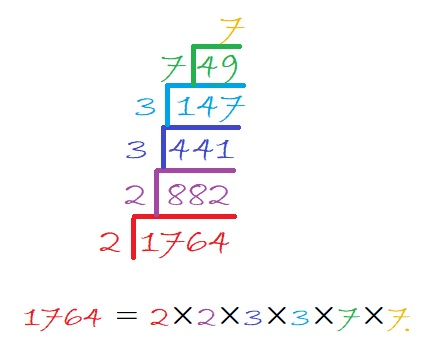• 1764 is a composite number and a perfect square.
• Prime factorization: 1764 = 2 × 2 × 3 × 3 × 7 × 7, which can be written 1764 = 2² × 3² × 7².
• 1764 has at least one exponent greater than 1 in its prime factorization so √1764 can be simplified. √1764 = √(42 × 42) = 42.
• The exponents in the prime factorization are 2, 2, and 2. Adding one to each exponent and multiplying we get (2 + 1)(2 + 1)(2 + 1) = 3 × 3 × 3 = 27. Therefore 1764 has exactly 27 factors. (Only perfect squares can have an odd number of factors.)
• The factors of 1764 are outlined with their factor pair partners in the graphic below.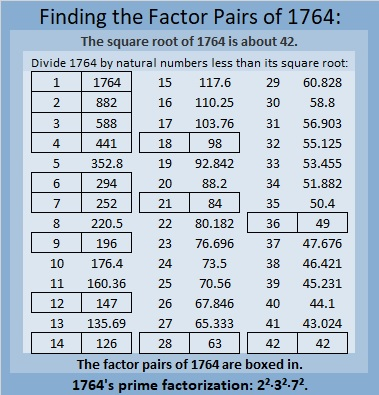### More About the Number 1764:

Square number, 1764,  looks square in some other bases, too:
It’s 900 in base 14 because 9(14²) + 0(14) + 0(1) = 1764,
484 in base 20 because 4(20²) + 8(20) + 4(1) = 1764,
400 in base 21 because 4(21²) + 0(21) + 0(1) = 1764,
169 in base 39 because 1(39²) + 6(39) + 9(1) = 1764,
144 in base 40 because 1(40²) + 4(40) + 4(1) = 1764,
121 in base 41 because 1(41²) + 2(41) + 1(1) = 1764, and
100 in base 42 because 1(42²) + 0(42) + 0(1) = 1764.

# When Does 1756 Look a Little Like π?

### Today’s Puzzle:

Here are 1756 tiny squares. Solve for n and you will know which centered pentagonal number 1756 is. You could also count the pentagons from the center outward.What triangular number multiplied by 5 is one less than 1756?

How do you pronounce pentagonal? Here’s a quick video on its correct pronunciation:

Confession: I had been mispronouncing all of those terms, but not anymore!

### Factors of 1756:

• 1756 is a composite number.
• Prime factorization: 1756 = 2 × 2 × 439, which can be written 1756 = 2² × 439.
• 1756 has at least one exponent greater than 1 in its prime factorization so √1756 can be simplified. Taking the factor pair from the factor pair table below with the largest square number factor, we get √1756 = (√4)(√439) = 2√439.
• The exponents in the prime factorization are 2 and 1. Adding one to each exponent and multiplying we get (2 + 1)(1 + 1) = 3 × 2 = 6. Therefore 1756 has exactly 6 factors.
• The factors of 1756 are outlined with their factor pair partners in the graphic below.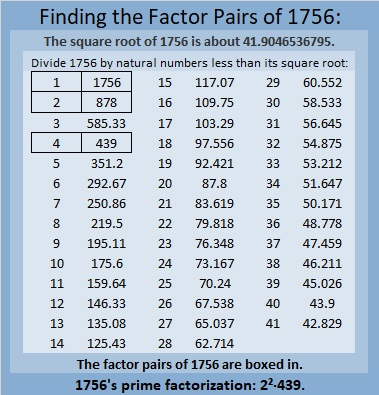### More About the Number 1756:

The centered pentagon above doesn’t really look like π, so when does 1756 look a little bit like π? Pi day in America is next week, so this is a time-sensitive question.

Actually,1756 looks a little like 10π in base 24. You see 1756₁₀ is the same as 314₂₄.
Why? Because 3(24²) + 1(24) + 4(1) = 1756.

1756₁₀ also is the same as 1024₁₂.
Why? Because 1(12³) + 0(12²) + 2(12) + 4(1) = 1756.
I think that’s cool because 2¹º = 1024.

1756 is the difference of two squares:
440² – 438² = 1456.

# 1755 Searching for Prime Decades

### Today’s Puzzle:

Charles Sanders got me thinking about prime decades and how to find them. What a great puzzle for me to work on!

A prime decade is a set of four prime numbers that look the same except for their last digit.

The first decade has 4 prime numbers, 2, 3, 5, 7, but it is NOT a prime decade because 1 and 9 are not prime numbers. 5, 7, 11, 13 is the only prime quadruplet that is not also a prime decade. The first prime decade is 11, 13, 17, 19.

Could 1751, 1753, 1757, and 1759 form a prime decade? Since 1755 is divisible by 15, none of those other four odd numbers can be divisible by 3 or 5, so it is worth looking into if they are all prime numbers. We don’t have to test all four numbers, however, we only need to test 1759 using modular arithmetic. If we note the remainder when we divide 1759 by each prime number from 7 to 41, we will know. (We only have to go to 41 because √1759 < 43, so we don’t need any prime number divisor greater than or equal to 43.)

Will any remainder be a red flag? Yes, if any remainder is 0, 2, 6, or 8, then one of those four odd numbers won’t be prime. Also, if we divide 1759 by 7 and get a remainder of 1, then 1758 and 1751 will both be divisible by 7, and we won’t have a prime decade.

I did the divisions and everything looked great except
1759 ÷ 7 has a remainder of 2, so 1759 – 2 or 1757 is divisible by 7, and
1759 ÷ 17 has a remainder of 8, so 1759 – 8 or 1751 is divisible by 17.

In general, to find prime decades we only need to test the last number of the decades and we only need to test decades if the last number minus 4 is divisible by 15. I made a chart showing only those last numbers, and they appear in the first column. The top two rows are the prime number divisors and their squares. We will divide the last number in the decades by the prime numbers whose square is less than that last number. The remainders in pink are red flags. That would be a 1 in the 7’s column, and 0, 2, 6, or 8 in any column. Any of those would disqualify a decade from being a prime decade. 🙁

The green last numbers (in the first column) show the last number of actual prime decades. 🙂
The yellow last numbers have only ONE divisor that disqualifies the decade. 😐

What are the prime decades in this first millennia?As you can see, decades ending in 19, 109, 199, and 829 are all prime decades. This chart can also be useful in finding prime triplets because at least two of the prime numbers in a prime triplet whose first prime is greater than 10 must come from a decade whose middle number is divisible by 15. If a decade has two prime triplets in it that meet that qualification, it is also a prime decade.

In the next graphic, we see that the decades ending in 1489 and 1879 are prime decades. Here we also test the decade in which our post number, 1755, appears. We see 1759 has remainders of 2 and 8, confirming that 1757 and 1751 are not prime numbers.There is only one prime decade, 2081, 2083, 2087, 2089, in the next set of decades, and only one other decade that even came close to being a second one.There are two prime decades in the next group and several close calls:None in the next group, but there are several decades that ALMOST qualified.There’s a prime decade is in this group as well as a handful of decades that almost qualified.In this next group, there are only two decades that even came close to qualifying.The next two millennia have no prime decades but they do have several decades that came close to qualifying.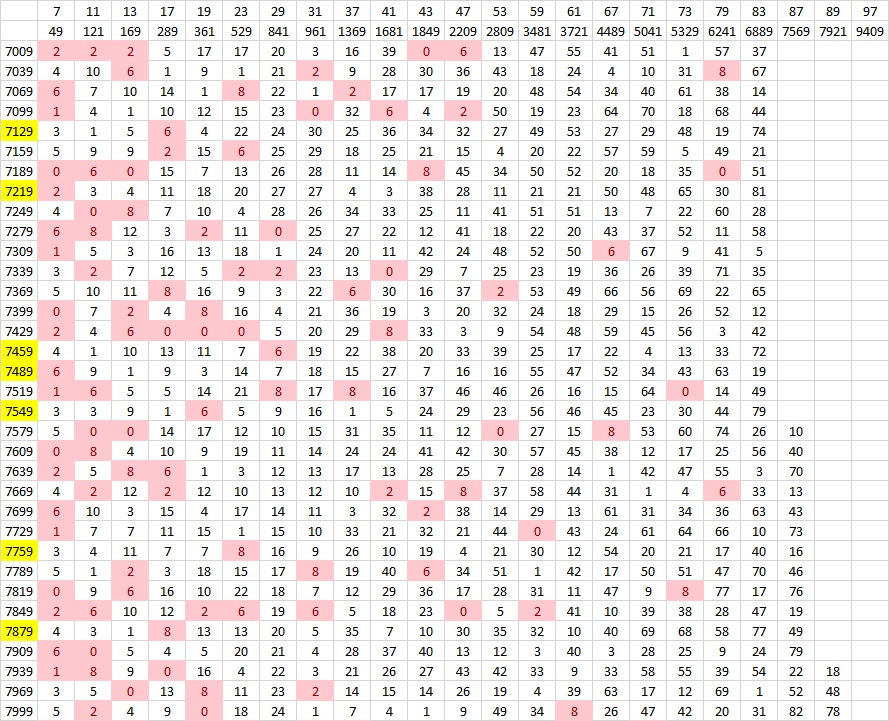Finally, we get another prime decade in this next group! (We would have had one right away if only 9047 wasn’t divisible by 83.)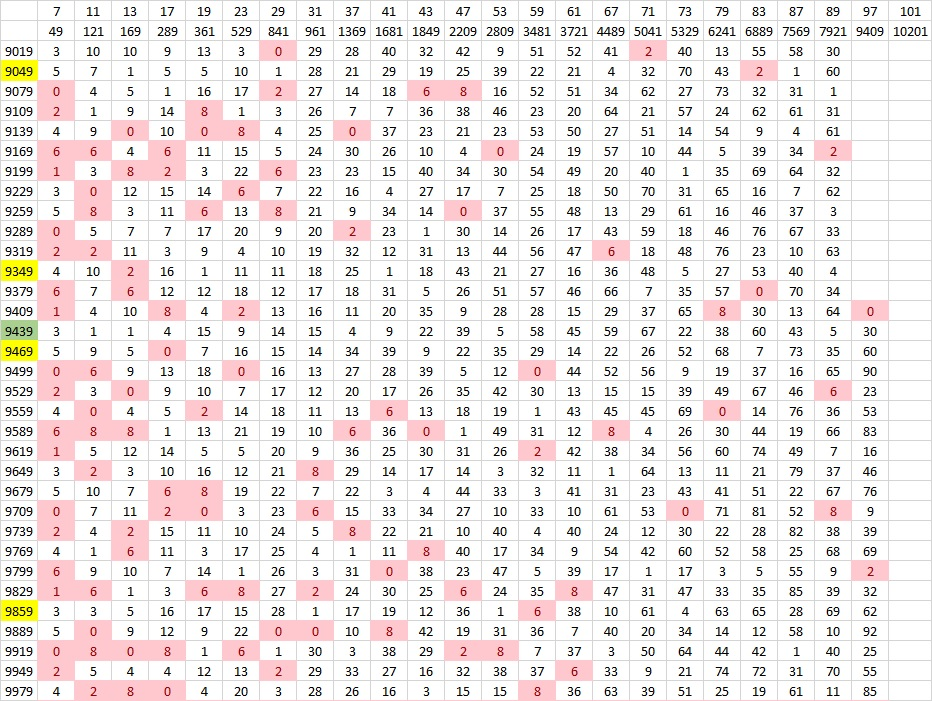I could go on literally forever, but I’ll stop now. Instead, I’ll write a little more about the post number, 1755.

### Factors of 1755:

• 1755 is a composite number.
• Prime factorization: 1755 = 3 × 3 × 3 × 5 × 13, which can be written 1755 = 3³ × 5 × 13.
• 1755 has at least one exponent greater than 1 in its prime factorization so √1755 can be simplified. Taking the factor pair from the factor pair table below with the largest square number factor, we get √1755 = (√9)(√195) = 3√195.
• The exponents in the prime factorization are 3,1 and 1. Adding one to each exponent and multiplying we get (3 + 1)(1 + 1) (1 + 1) = 4 × 2 × 2 = 16. Therefore 1755 has exactly 16 factors.
• The factors of 1755 are outlined with their factor pair partners in the graphic below.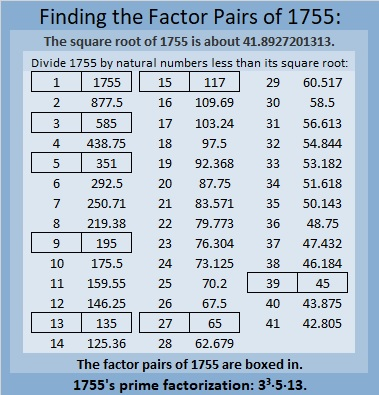### More About the Number 1755:

1755 is the hypotenuse of FOUR Pythagorean triples:
432-1701-1755, which is 27 times (16-63-65)
675-1620-1755, which is (5-12-13) times 135,
891-1512-1755, which is 27 times (33-56-65), and
1053-1404-1755, which is (3-4-5) times 351.

# 1754 and a Level 2 Puzzle

### Today’s Puzzle:

Can you write each number from 1 to 12 in the first column and again in the top row so that the products of the numbers you write are the given clues?### Factors of 1754:

• 1754 is a composite number.
• Prime factorization: 1754 = 2 × 877.
• 1754 has no exponents greater than 1 in its prime factorization, so √1754 cannot be simplified.
• The exponents in the prime factorization are 1 and 1. Adding one to each exponent and multiplying we get (1 + 1)(1 + 1) = 2 × 2 = 4. Therefore 1754 has exactly 4 factors.
• The factors of 1754 are outlined with their factor pair partners in the graphic below.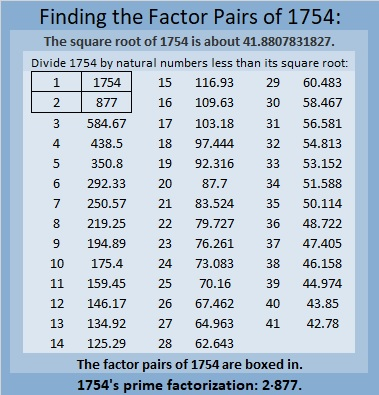### More About the Number 1754:

1754 is the sum of two squares:
35² + 23² = 1754.

1754 is the hypotenuse of a Pythagorean triple:
696-1610-1754, calculated from 35² – 23², 2(35)(23), 35² + 23².
It is also 2 times (348-805-877).

# 1753 What Is the Slope of the Driveway?

### Today’s Puzzle:

With so much snow all around it is easy to think about summer. Last summer my family and I stayed at a magnificent Airbnb. There was more than enough room for my husband and me, our children and their spouses, and our grandchildren (32 people in total).

The owners of the Airbnb wrote that the best views come from homes with steep driveways. I will share some of those views later in the post. But just how steep was the driveway? I took a picture and added a grid so you can calculate the slope. Notice that the driveway zigzags. You can estimate where the front tire of the van parked at the top of the driveway hits the pavement to calculate the slope of the top part of the driveway.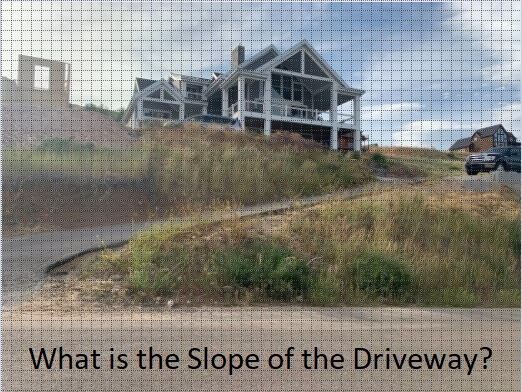Here’s the top half of the driveway:

###The beautiful view from the top of the driveway:

###The next three pictures are gorgeous views from inside the house or from a balcony:

###The views definitely made the steep driveway worth it!

Since this is my 1753rd post, I’ll write a little about that number.

### Factors of 1753:

• 1753 is a prime number.
• Prime factorization: 1753 is prime.
• 1753 has no exponents greater than 1 in its prime factorization, so √1753 cannot be simplified.
• The exponent in the prime factorization is 1. Adding one to that exponent we get (1 + 1) = 2. Therefore 1753 has exactly 2 factors.
• The factors of 1753 are outlined with their factor pair partners in the graphic below.How do we know that 1753 is a prime number? If 1753 were not a prime number, then it would be divisible by at least one prime number less than or equal to √1753. Since 1753 cannot be divided evenly by 2, 3, 5, 7, 11, 13, 17, 19, 23, 29, 31, 37, or 41, we know that 1753 is a prime number.

### More About the Number 1753:

1753 is a powerful sum:
2¹º + 3⁶ = 1753.

Since both of those exponents are even, 1753 is also the sum of two squares:
32² + 27² = 1753.

1753 is the hypotenuse of a Pythagorean triple:
295-1728-1753, calculated from 32² – 27², 2(32)(27), 32² + 27².

Here’s another way we know that 1753 is a prime number: Since its last two digits divided by 4 leave a remainder of 1, and 32² + 27² = 1753 with 32 and 27 having no common prime factors, 1753 will be prime unless it is divisible by a prime number Pythagorean triple hypotenuse less than or equal to √1753. Since 1753 is not divisible by 5, 13, 17, 29, 37, or 41, we know that 1753 is a prime number.

# 1746 Love-Struck with Multiplication

### Today’s Puzzle:

If you solve this Valentine’s multiplication table puzzle, you might just become love-struck with multiplication! It’s a level 6 puzzle so you might find most of the clues to be tricky. Be sure to use logic as you place each number from 1 to 10 in both the first column and also in the top row. There is only one solution.### Factors of 1746:

• 1746 is a composite number.
• Prime factorization: 1746 = 2 × 3 × 3 × 97, which can be written 1746 = 2 × 3² × 97.
• 1746 has at least one exponent greater than 1 in its prime factorization so √1746 can be simplified. Taking the factor pair from the factor pair table below with the largest square number factor, we get √1746 = (√9)(√194) = 3√194.
• The exponents in the prime factorization are 1, 2, and 1. Adding one to each exponent and multiplying we get (1 + 1)(2 + 1)(1 + 1) = 2 × 3 × 2 = 12. Therefore 1746 has exactly 12 factors.
• The factors of 1746 are outlined with their factor pair partners in the graphic below.### More About the Number 1746:

Why is 1746 the sum of two squares? We can tell by looking at its PRIME factors:
2 is a power of 2,
3² is an even power, and
97 is a prime Pythagorean hypotenuse. (It is prime and one more than a positive multiple of 4).

At least one of its prime factors is a Pythagorean triple hypotenuse, and ALL of the rest of its non-Pythagorean-hypotenuse prime factors are either a power of 2 or combine to make a perfect square. Thus, 1746 is the sum of two squares. What are the two squares?
39² + 15² = 1746.

1746 is the hypotenuse of a Pythagorean triple:
1170-1296-1746, calculated from 2(39)(15), 39² – 15², 39² + 15².
It is also 18 times (65-72-97).

# 1743 Finding Ways to Transform My Heart

### Today’s Puzzle:

An equation of a unit circle centered at the origin is x² + y² = 1.

If we change just the “y” part of that equation, we can get a lovely heart just in time for Valentine’s Day. Try it yourself by typing the equations into Desmos.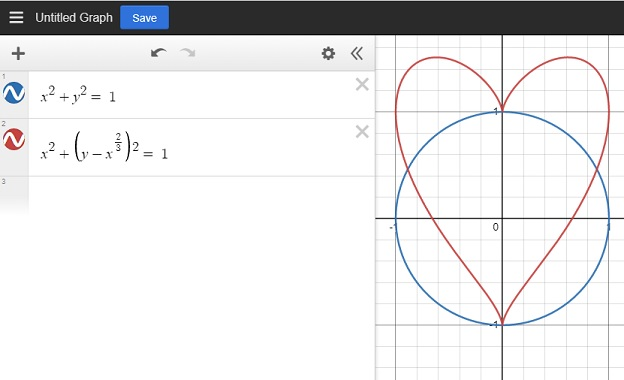There are other mathematical equations for a heart, but this is the one I’m exploring in this post.

I was puzzled over how I could transform that heart. Can I make it bigger, or dilate it? Can I slide it away from the origin or translate it? Can I rotate it? Can I reflect it across the x or y-axis?

These are questions I’d like you to explore as well.

### Heart Dilation:

In this first graphic, I was able to make my heart bigger. What kind of math let me do that? Also, how did I color the inside of some of the hearts? Look at the equations next to the heart and try to figure it out. The concentric hearts are evenly spaced. Do you recognize a pattern in the numbers that made that happen?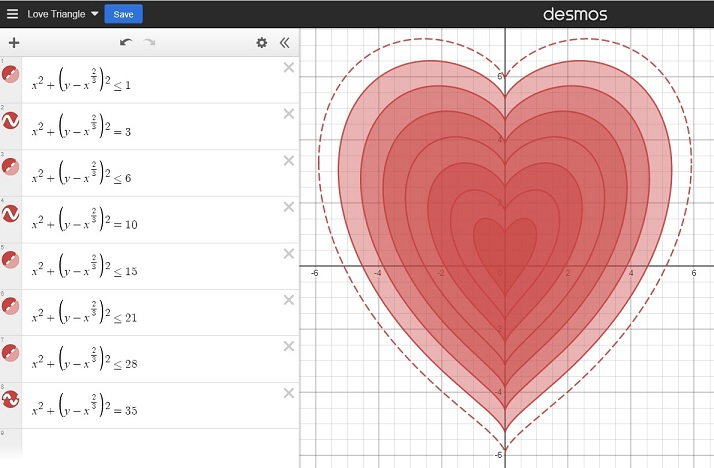### Heart Slide (Translation):

If we changed the center of a circle to (a, -b) instead of the origin, we would slide the whole circle. Here’s how we change the equation of the circle to give it a new center:
(x-a)²+(y+b)² = 1.

Similarly, in the next graphic, I was able to slide my heart away from the origin. How did I do that? Look at the equations to see how.### Heart Rotation:

A circle looks the same no matter how it is rotated, but the same isn’t true for a heart. Look at the equations below. How was I able to rotate my heart around the origin?### Heart Reflection:

Since a heart is symmetric, its reflection across the x-axis doesn’t look that interesting to me. Instead, I created a double heart that I reflected across both the x-axis and the y-axis: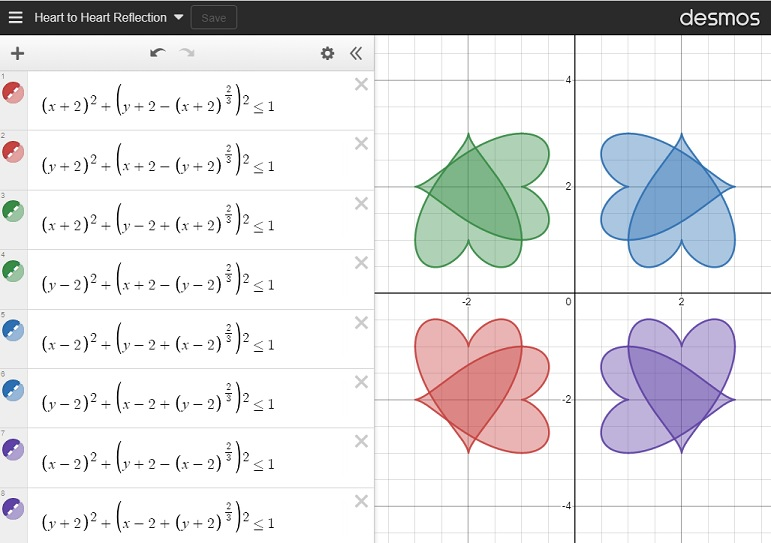### Just for Fun:

Next, I was curious about what would happen if I changed the exponents on the outside of the parenthesis, so I changed a 2 from my original equation to an 8 in a couple of different places as I moved the heart from left to right. How did changing the exponent affect my heart? I found that as long as the exponent stays even, it still looks a little like a heart.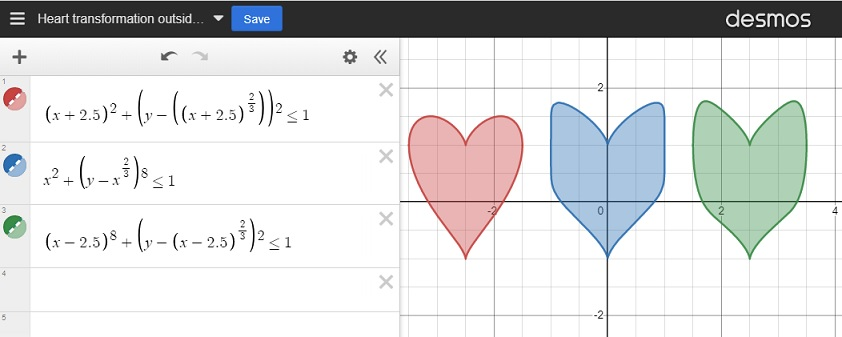I was also curious about what would happen to my heart if I changed the “2/3” to a different fraction. I used fractions less than one as well as fractions greater than one. For many of my fractions, I used the post number, 1743, as the denominator. As long as the numerator was even and the denominator was odd, the graph still looked mostly like a heart. However, the closer the fraction was to zero, the more it looked like a circle.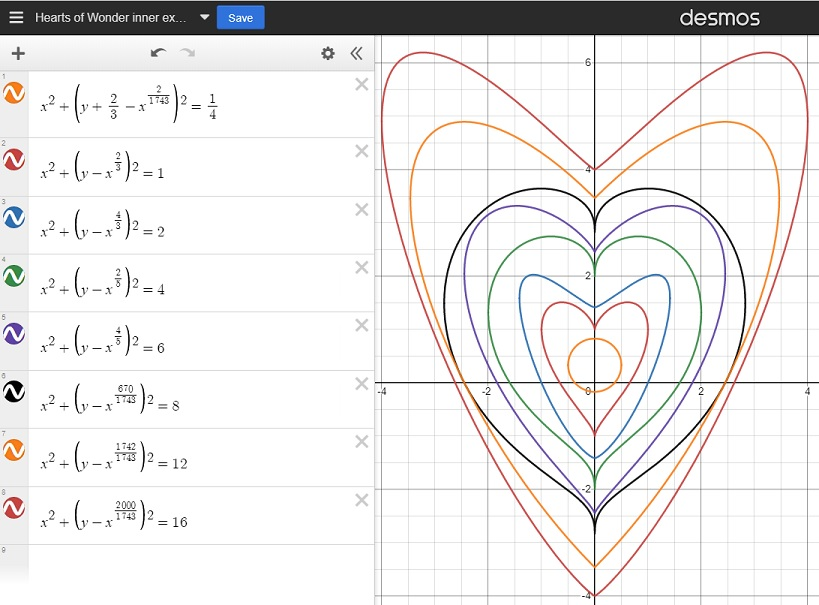Finally, I created this lovely flower using some of what I learned by making these transformations:And for just a little bit more fun, I created a simple but chaotic-looking animation that I’ve titled Hearts in Motion. Enjoy!

I had so much fun exploring this heart in Desmos. Thank you for allowing me to share my excitement with you. Here’s a different Desmos heart created by a reader and shared with me. Click on it to make the heart beat:

### Factors of 1743:

• 1743 is a composite number.
• Prime factorization: 1743 = 3 × 7 × 83.
• 1743 has no exponents greater than 1 in its prime factorization, so √1743 cannot be simplified.
• The exponents in the prime factorization are 1, 1, and 1. Adding one to each exponent and multiplying we get (1 + 1)(1 + 1)(1 + 1) = 2 × 2 × 2 = 8. Therefore 1743 has exactly 8 factors.
• The factors of 1743 are outlined with their factor pair partners in the graphic below.### More About the Number 1743:

1743 is the difference of two squares in four different ways:
872² – 871² = 1743,
292² – 289² = 1743,
128² – 121² = 1743, and
52² – 31² = 1743.

# 1741 What Do You Notice About the Remainders?

### Today’s Puzzle:

The table below is a list of the first 280 prime numbers. Below each prime number is its remainder when the prime number is divided by 6. What do you notice about the remainders? What do you wonder?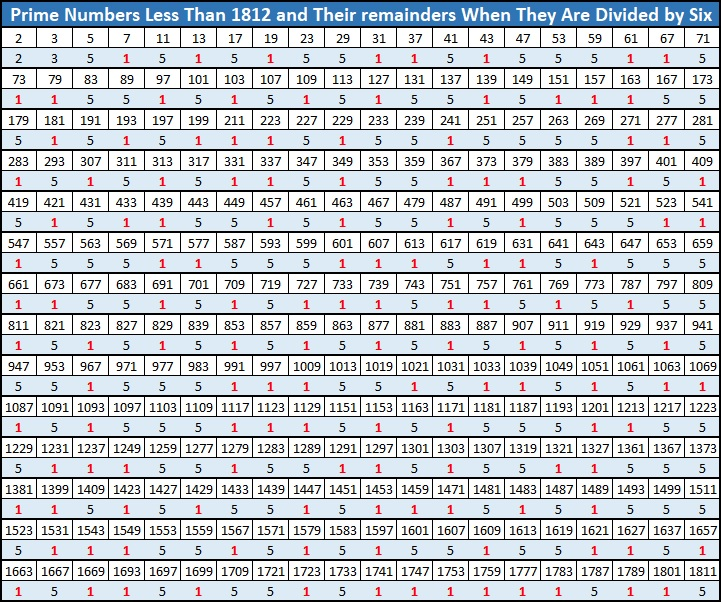Did you notice a fun fact starting with the number 1741? I would like to thank OEIS.org for making me aware of it.

### Factors of 1741:

• 1741 is a prime number.
• Prime factorization: 1741 is prime.
• 1741 has no exponents greater than 1 in its prime factorization, so √1741 cannot be simplified.
• The exponent in the prime factorization is 1. Adding one to that exponent we get (1 + 1) = 2. Therefore 1741 has exactly 2 factors.
• The factors of 1741 are outlined with their factor pair partners in the graphic below.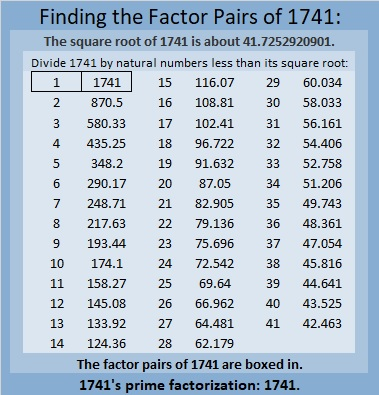How do we know that 1741 is a prime number? If 1741 were not a prime number, then it would be divisible by at least one prime number less than or equal to √1741. Since 1741 cannot be divided evenly by 2, 3, 5, 7, 11, 13, 17, 19, 23, 29, 31, 37, or 41, we know that 1741 is a prime number.

### More About the Number 1741:

1741 is the sum of two squares:
30² + 29² = 1741.

Here’s another way we know that 1741 is a prime number: Since its last two digits divided by 4 leave a remainder of 1, and 30² + 29² = 1741 with 30 and 29 having no common prime factors, 1741 will be prime unless it is divisible by a prime number Pythagorean triple hypotenuse less than or equal to √1741. Since 1741 is not divisible by 5, 13, 17, 29, 37, or 41, we know that 1741 is a prime number.

Since 29 and 30 are consecutive numbers, we have another fun fact: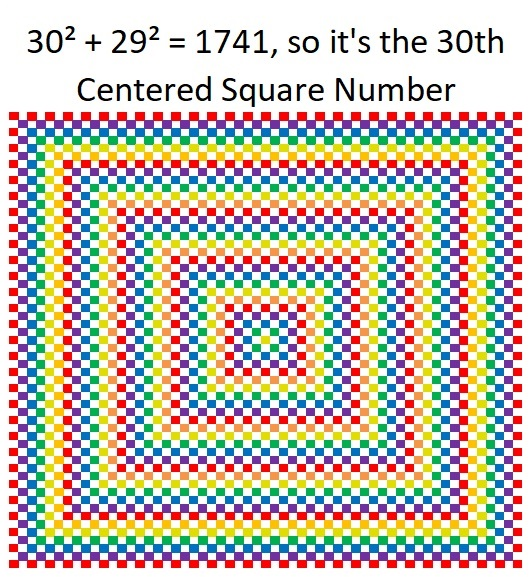And 1741 is the hypotenuse of a Pythagorean triple:
59-1740-1741, calculated from 30² – 29², 2(30)(29), 30² + 29².

1741 is also the difference of two consecutive squares:
871² – 870² = 1741.

# 1737 What Logic Will You Use to Solve This Puzzle?

### Today’s Puzzle:

Place each number from 1 to 12 in both the first column and the top row so that those numbers are the factors of the given clues. This is a level 6 puzzle so the logic needed to solve the puzzle will be a little more complicated. Good luck!### Factors of 1737:

1 + 7 + 3 + 7 = 18, a number divisible by nine, so 1718 is divisible by 9.

• 1737 is a composite number.
• Prime factorization: 1737 = 3 × 3 × 193, which can be written 1737 = 3² × 193.
• 1737 has at least one exponent greater than 1 in its prime factorization so √1737 can be simplified. Taking the factor pair from the factor pair table below with the largest square number factor, we get √1737 = (√9)(√193) = 3√193.
• The exponents in the prime factorization are 2 and 1. Adding one to each exponent and multiplying we get (2 + 1)(1 + 1) = 3 × 2 = 6. Therefore 1737 has exactly 6 factors.
• The factors of 1737 are outlined with their factor pair partners in the graphic below.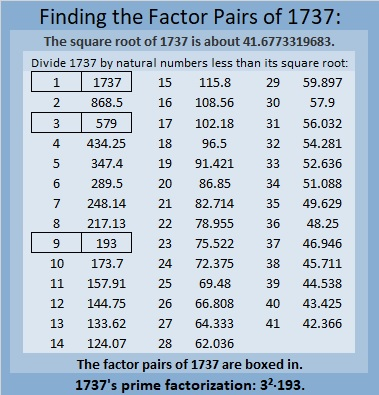### More About the Number 1737:

1737 is the difference of two squares in three ways:
869² – 868² = 1737,
291² – 288² = 1737, and
101² – 92² = 1737.

1737 is the sum of consecutive numbers:
868 + 869 = 1737,
578 + 579 +560 = 1737,
287 + 288 + 289 + 290 + 291 + 292 = 1737,
189 + 190 + 191 + 192 + 193 + 194 + 195 + 196 + 197 = 1737, and
88+89+90+91+92+93+94+95+96+97+98+99+100+101+102+103+104+105=1737.

That first sum uses the same numbers as the first difference of two squares. Check out these square equations!

292² – 291² + 290² – 289² + 288² – 287² = 1737.

105² – 104² + 103² – 102² + 101² – 100² + 99² – 98² + 97² – 96² + 95² – 94² + 93² – 92² + 91² – 90² + 89² – 88² = 1737.

(But since 101² – 92² = 1737, we can conclude that all the rest of the numbers added and subtracted will give us zero!)

1737 is also the sum of two squares:
36² + 21² = 1737.

1737 is the hypotenuse of a Pythagorean triple:
855-1512-1737 calculated from 36² – 21², 2(36)(21), 36² + 21².
That triple is also 9 times (95-168-193).

That Pythagorean triple means that
855² + 1512² = 1737².

From OEIS.org, we learn that when we add up these three consecutive squares,
1736² + 1737² + 1738² we get a palindrome, specifically, 9051509. Cool!

1737² = 1736² + 1734² – 1733² + 1732² – 1731² – 1729² + 1728².